## 1. What is the slope of the line through the points (-2, -1) and (8, -3)?

Question

1.
What is the slope of the line through the points (-2, -1) and (8, -3)?

in progress 0
3 months 2021-08-12T23:05:15+00:00 1 Answers 2 views 0

The slope of the line is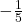in fraction form or -0.2 in decimal form.

Step-by-step explanation:

To solve for the slope of the line, use the rise over run formula. The rise over run formula is a measure of how much something changes vertically compared to how much it changes in the horizontal direction. The rise over run formula calculates the difference between the two points in the vertical direction (rise) and then divide it by the difference in the horizontal direction (run). The formula looks like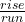, but in terms of finding a slope, it looks like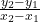.

For finding the slope of this line with the points (-2,-1) and (8,-3), start by writing down the information given from the question.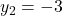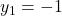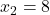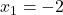Next, plug in the information given from the question into the formula, and the formula will look like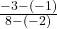. Then, simplify the equation, which will look like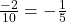. The final answer will bein fraction form or -0.2 in decimal form.